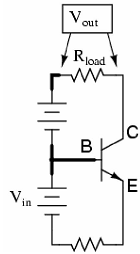## Explain common base configuration, Electrical Engineering

Assignment Help:

Q. Explain common base configuration?

Common-base transistor amplifiers are so-called because the input and output voltage points share the base lead of the transistor in common with each other, not considering any power suppliesThe most striking characteristic of this configuration is that the input signal source must carry the full emitter current of the transistor. Because the input current exceeds all other currents in the circuit, including the output current, the current gain of this amplifier is actually less than 1 (notice how Rload is connected to the collector, thus carrying slightly less current than the signal source). In other words, it attenuates current rather than amplifying it. In the common-base circuit, we follow basic transistor parameter: the ratio between collector current and emitter current, which is a fraction always less than 1. This fractional value for any transistor is called the alpha ratio, or I ratio.

#### Parking lot project, i dont know how to start

i dont know how to start

#### Communication, can I get matlab code for out of band radiation reduction of...

can I get matlab code for out of band radiation reduction ofdm system

#### Transformers, A 15kVA has a turns ratio 1:10. If the voltage applied is 13....

A 15kVA has a turns ratio 1:10. If the voltage applied is 13.8kV and the applied load is 20+j6. Find the current in the secondary winding and the active and reactive power consumed

#### 2365-305 Electrical systems design TASK A, Hi, I need help with this assign...

Hi, I need help with this assignment. Is this assignment something you can help me with?

#### BE, WHICH PROJECT WILL BE BEST THESE DAY

WHICH PROJECT WILL BE BEST THESE DAYS

what is emf

#### Digital electronic, What are the advantages of wired-AND connection of digi...

What are the advantages of wired-AND connection of digital IC

#### Determine maximum length for cable, You have been asked to help on the desi...

You have been asked to help on the design of CDU's new electric vehicle. The vehicle is to be powered by a 96V, 300A battery pack and you need to decide on the maximum length you c

#### Example of binary subtraction of negative number , Example of binary subtra...

Example of binary subtraction of Negative number Case 2.   Negative number is more than  the positive number ( i e, answer  is negative)  Example : Subtract 92 10 ( 101

#### States the superposition theorem, The superposition theorem states: 'In...

The superposition theorem states: 'In any network made up of linear resistances and having more than single source of e.m.f, the resultant current flowing in any branch is the